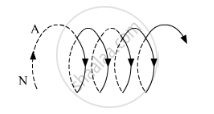Share

# An Observer to the Left of a Solenoid of N Turns Each of Cross Section Area 'A' Observes that a Steady Current I in It Flows in the Clockwise Direction. Depict the Magnetic Field Lines Due to the Solenoid Specifying Its Polarity and Show that It Acts as a Bar Magnet of Magnetic Moment M = NIA - CBSE (Science) Class 12 - Physics

ConceptSolenoid and the Toroid - the Solenoid

#### Question

An observer to the left of a solenoid of N turns each of cross section area 'A' observes that a steady current I in it flows in the clockwise direction. Depict the magnetic field lines due to the solenoid specifying its polarity and show that it acts as a bar magnet of magnetic moment m = NIA.#### Solution

Given that the current flows in the clockwise direction for an observer on the left side of the solenoid. This means that left face of the solenoid acts as south pole and right face acts as north pole. Inside a bar magnet, the magnetic field lines are directed from south to north. Therefore, the magnetic field lines are directed from left to right in the solenoid.

Magnetic moment of single current carrying loop is given by

m'=IA

where

I = Current flowing through the loop
A = area of the loop

So, magnetic moment of the whole solenoid is given by

m=Nm'=N(IA)

Is there an error in this question or solution?

#### Video TutorialsVIEW ALL 

Solution An Observer to the Left of a Solenoid of N Turns Each of Cross Section Area 'A' Observes that a Steady Current I in It Flows in the Clockwise Direction. Depict the Magnetic Field Lines Due to the Solenoid Specifying Its Polarity and Show that It Acts as a Bar Magnet of Magnetic Moment M = NIA Concept: Solenoid and the Toroid - the Solenoid.
S